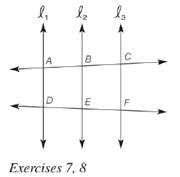Chapter 5.6, Problem 8EElementary Geometry For College St...

7th Edition
Alexander + 2 others
ISBN: 9781337614085

Solutions

Chapter
SectionElementary Geometry For College St...

7th Edition
Alexander + 2 others
ISBN: 9781337614085
Textbook Problem

Given: l 1 ∥ l 2 ∥ l 3 , A B = 5 , B C = x , D E = x − 2 , E F = 7 Find: x , B C , D ETo determine

To find:

x,BC,DE.

Explanation

Given:

l1l2l3 and AB=5,BC=x,DE=x2,EF=7.

Theorem used:

When three (or more) parallel lines are cut by a pair of transversals, the transversals are divided proportionally by the parallel lines.

Calculation:

Given: l1l2l3

Since when three (or more) parallel lines are cut by a pair of transversals, the transversals are divided proportionally by the parallel lines.

ABDE=BCEF

Also given that AB=5,BC=x,DE=x2,EF=7.

Thus 5x2=x7.

Now let us find the value of x.

Still sussing out bartleby?

Check out a sample textbook solution.

See a sample solution

The Solution to Your Study Problems

Bartleby provides explanations to thousands of textbook problems written by our experts, many with advanced degrees!

Get Started

In Exercises 1-4, simplify the expression. x2+6x+8x26x16

Calculus: An Applied Approach (MindTap Course List)

Evaluate the integral. 35. /2/2x1+cos2xdx

Single Variable Calculus: Early Transcendentals

True or False: These lines are skew:

Study Guide for Stewart's Multivariable Calculus, 8th

True or False: n=1an converges if and only if limnan=0.

Study Guide for Stewart's Single Variable Calculus: Early Transcendentals, 8th